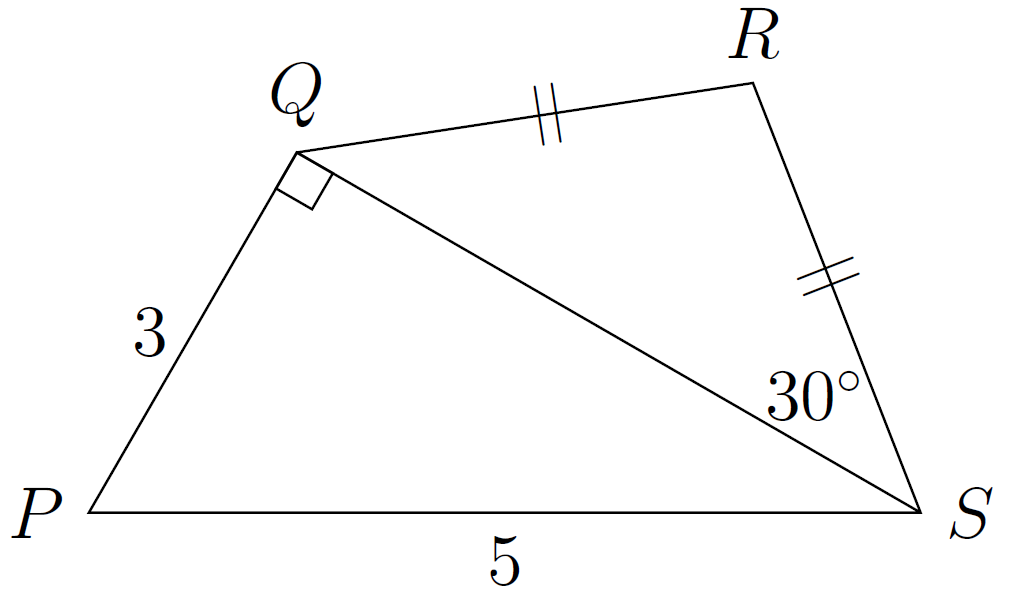## amc-2014-sr-15

In the diagram, $$PS=5 %speech% ,P S equals 5,$$, $$PQ=3 %speech% ,P Q equals 3,$$, $$\triangle PQS %speech% ,triangle P Q S,$$ is right-angled at $$Q$$, $$\angle QSR=30^\circ %speech% ,angle Q S R is 30 degrees,$$ and $$QR=RS %speech% ,Q R equals R S,$$.The length of $$RS$$ is